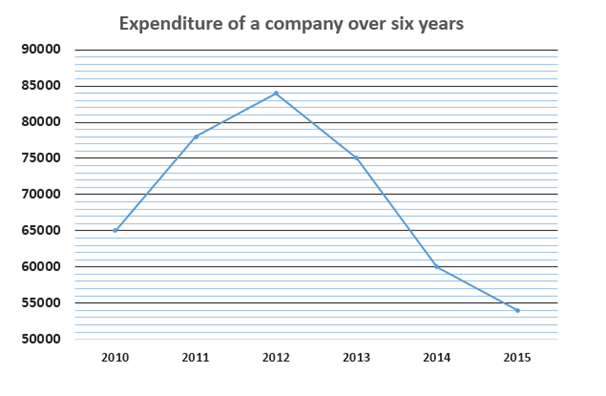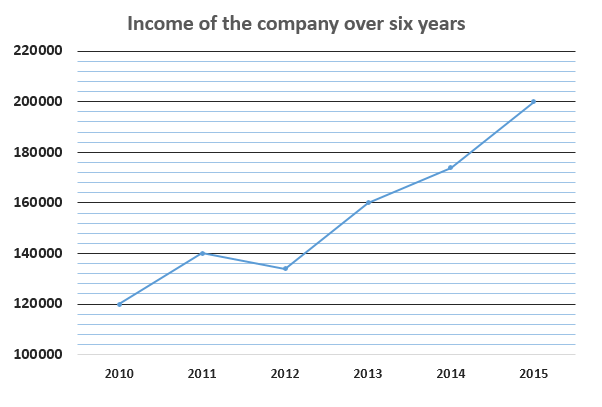# IBPS RRB PO Mains Quantitative Aptitude Questions 2019 (Day-01)

Dear Aspirants, Our IBPS Guide team is providing new series of Quantitative Aptitude Questions for IBPS RRB PO Mains 2019 so the aspirants can practice it on a daily basis. These questions are framed by our skilled experts after understanding your needs thoroughly. Aspirants can practice these new series questions daily to familiarize with the exact exam pattern and make your preparation effective.

Check here for IBPS RRB PO Mains Mock Test 2019

### Click Here to Subscribe Crack High Level Puzzles & Seating Arrangement Questions PDF 2019 Plan

[WpProQuiz 6791]

Directions (1 – 5): Study the following information carefully and answer the questions given below:

The following line graph shows the income (in Rs.) and expenditure (in Rs.) of a company over six years.1) If the expenditure of the company in 2016 is 20% more than the expenditure of the company in 2015 and income in 2016 is 20% less the income in 2015, find the percent profit earned by the company in 2016.

a) 112.12%

b) 129.99%

c) 146.91%

d) 133.33%

e) None of these

2) Find the respective ratio of the expenditure of the company in 2011 and 2013 together and income of the company in the same years.

a) 131:156

b) 51:100

c) 149:289

d) 119:234

e) None of these

3) Profit earned by the company in 2010 is what percent more/less than the profit earned by the company in 2012?

a) 10%

b) 18%

c) 15%

d) 20%

e) None of these

4) Find the difference between expenditure of the company in all the years together and income of the company in all the years together.

a) Rs.142400

b) Rs.362400

c) Rs.512000

d) Rs.468200

e) None of these

5) Find the approximate percent increase in the percent profit earned by the company from 2010 to 2015.

a) 154%

b) 289%

c) 180%

d) 110%

e) 218%

6) Raju, Rohan, Sohan and Vinay entered into a partnership with investment in the ratio 7: 6: 5: 8. After one year, Raju discontinued and Sohan doubled his investment. After one more year, Rohan and Vinay withdrew half of their investment amount. At the end of three years, they earned a profit of Rs.268000. Find the difference between the shares of Raju and Vinay in the profit.

a) Rs.64000

b) Rs.52000

c) Rs.48000

d) Rs.56000

e) None of these

7) Seema can complete 50% of a work in 10 days. Reeju can complete 25% of the work in 4 days and Sanju can complete the whole work in 12 days. If Seema and Reeju worked for 5 days and left, in how many days Sanju can complete the remaining work?

a) 23/4 days

b) 21/4 days

c) 19/6 days

d) 17/4 days

e) None of these

8) Three poles A, B and C are in a straight line such that B is equidistant from A and C. A boat can go from A to C downstream and returns to B upstream in 22 hours. If the speed of the boat in still water is 8 km/h and speed of the stream is 2 km/h, find the distance between A and C.

a) 80 km

b) 75 km

c) 60 km

d) 55 km

e) None of these

9) Bag P contains 4 white balls and bag Q contains 6 green balls. Some balls are transferred from bag Q to bag P. Probability of drawing 2 white balls from bag P is 3/14. Find the number of balls transferred to bag P.

a) 2

b) 4

c) 6

d) 3

e) None of these

10) A road of width 7m is running around a circular field from outside. Diameter of the circular field is 28m. Find the cost of fencing the road at the rate of Rs.16/m2.

a) Rs.18564

b) Rs.22456

c) Rs.12986

d) Rs.22176

e) None of these

Direction (1-5) :

Expenditure of the company in 2016 = 54000 x 120/100 = Rs.64800

Income of the company in 2016 = 200000 x 80/100 = Rs.160000

Profit % = (160000 – 64800)/64800 x 100

= 95200/64800 x 100

= 146.91%

Expenditure of the company in 2011 and 2013 together = 78000 + 75000 = Rs.153000

Income of the company in 2011 and 2013 together = 140000 + 160000 = Rs.300000

Required ratio = 153000: 300000

= 153: 300 =51: 100

Profit earned by the company in 2010 = 120000 – 65000 = Rs.55000

Profit earned by the company in 2012 = 134000 – 84000 = Rs.50000

Required percentage = (55000 – 50000)/50000 x 100

= 5000/50000 x 100

= 10%

Expenditure of the company in all the years together = 65000 + 78000 + 84000 + 75000 + 60000 + 54000

= Rs.416000

Income of the company in all the years together = 120000 + 140000 + 134000 + 160000 + 174000 + 200000

= Rs.928000

Required difference = 928000 – 416000

= Rs.512000

Percent profit earned by the company in 2010 = (120000 – 65000)/65000 x 100

= 55000/65000 x 100

= 84.61%

= 85% (approx.)

Percent profit earned by the company in 2015 = (200000 – 54000)/54000 x 100

= 146000/54000 x 100

= 270.37%

= 270% (approx.)

Percent increase = (270 – 85)/85 x 100

= 185/85 x 100

= 217.64%

= 218% (approx.)

Direction (6-10) :

Let the investment amount of Raju, Rohan, Sohan and Vinay be Rs.7x, Rs.6x, Rs.5x and Rs.8x respectively.

Ratio of shares in the profit:

Raju: Rohan: Sohan: Vinay = 7x: (6x + 6x + 3x): (5x + 10x + 10x): (8x + 8x + 4x)

= 7x: 15x: 25x: 20x

= 7:15:25:20

Required difference = (20 – 7)/67 x 268000

= 13/67 x 268000

= Rs.52000

Seema – 2 x 10 = 20 days

Reeju – 4 x 4 = 16 days

Sanju – 12 days

Let the required number of days = x

According to the question

5/20 + 5/16 + x/12 = 1

=> (20 + 25)/80 + x/12 = 1

=> 45/80 + x/12 = 1

=> x/12 = 1 – 9/16

=> x/12 = (16 – 9)/16

=> x/12 = 7/16

=> x = 7/16 x 12

=> x = 21/4 days

Let the distance between A and C is 2d.

According to the question

2d/(8 + 2) + d/(8 – 2) = 22

=> 2d/10 + d/6 = 22

=> d/5 + d/6 = 22

=>(6d + 5d)/30 = 22

=> 11d/30 = 22

=> d/30 = 2

=> d = 60 km

The distance between A and C = 2d = 120 km

Let the number of balls transferred = x

Now,

xc2/(4 + x)c2 = 3/14

=>x (x – 1)/(4 + x)(3 + x) = 3/14

=> 14x2 – 14x = 3 x (12 + 4x + 3x + x2)

=> 14x2 – 14x = 36 + 21x + 3x2

=> 11x2 – 35x – 36 = 0

=> (x – 4)(11x + 9) = 0

=> x = 4, -9/11(rejected)

=> x = 4

Area of the circular field = π(R2 – r2)

= 22/7 x (28 + 7)2 – 22/7 x (28)2

= 22/7 x (35)2 – 22/7 x (28)2

= 3850 – 2464

= 1386 m2

Cost of fencing = 16 x 1386 = Rs.22176